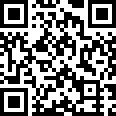Communicate with Supplier? SupplierMr. Sun Zhaohai
What can I do for you?

Home > About Us > Definitions and Terminology

Definitions and Terminology

Piezoelectric ceramics are, after firing, composed of small grains (crystallites), each containing domains in which the electric dipoles are aligned. These grains and domains are randomly oriented, so the net electric dipole is zero, i.e. the ceramics do not exhibit piezoelectric properties. The application of sufficiently strong D. C. field will orient the domains in the field direction, as nearly as the orientation of the crystal axes allows. This ability to change the orientation of the domains and achieve a net polarization is called ferroelectricity. A remanent polarization can be created in ferroelectric ceramics by polarization. After the poling process is complete, a voltage with the same polarity as the poling voltage causes expansion along the poling axis and contraction perpendicular to the poling axis. Compressive or tensile forces applied to the ceramic element will generate a voltage.

Definitions and Terminology

In piezoelectric ceramics, material characteristics depend on the direction of the applied field, displacement, stress and strain. Hence superscripts and subscripts indicating the direction are added to the symbols. The direction of polarization is generally designated as the z-axis of an orthogonal crystallographic system. The axes x, y and z are respectively represented as 1, 2 and 3 directions and the shear about these axes are represented as 4, 5 and 6. This is shown schematically on the Symbols and Terminoloy Chart. The various piezoelectric material constants are generally expressed with subscripts using this notation. In addition to the above, planar modes are sometimes expressed with a subscript 'p'. Superscripts indicate a constant mechanical or electrical boundary condition. The table below gives a general description of the superscripts.

Parameter Symbol Condition
Stress T Mechanically free
Field E Electrical short circuit
Displacement D Electrical open circuit

Strain S Mechanically clampled

Curie Temperature
The crystal structure of a material changes at the Curie temperature, Tc, from piezoelectric (non-symmetrical) to a non-piezoelectric (symmetrical) form. This phase change is accompanied by a peak in the dielectric constant and a complete loss of all piezoelectric properties.

Table of Symbols
A Surface area (m2)
c Stiffness coefficient (N/m2)
C Capacitance (F)
d Piezoelectric charge coefficient (C/N)
D Diameter (m)
f1, f2 -3dB points from the resonance frequency fr
fa Anti-resonance frequency (Hz)
fr Resonance frequency (HZ)
g Piezoelectric voltage coefficient (Vm/N)
k Coupling factor
K Relative dielectric constant
L Length (m)
N Frequency constant (Hz*m)
Qm Mechanical Q factor
s Elastic compliance (m2/N)
T Thickness (m)
Tc Curie temperature (oC)
W Width (m)
Y Young1s modulus (N/m2)
Zm Minimum impedance at fr (ohm)
tan δ Dissipation factor
εo Permittivity of free space (8.854x10-12F/m)
εT Permittivity (F/M)
ν Sonic velocity (m/s)
ρ Density (kg/m3)
σE Poisson's ratio

Send InquiryMr. Sun Zhaohai

Email:

ceo@zbyuhai.com

Send Inquiry

Tel:0086-533-4230012

Fax:

Mobile Phone:+8618753397607

Email:ceo@zbyuhai.com